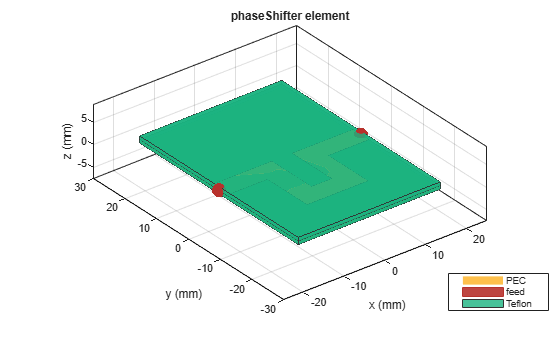# phaseShifter

Create phase shifter in microstrip form

## Description

Use the `phaseShifter` object to create a phase shifter in the microstrip form. Phase shifters change the phase of a signal using proper matching at the input and output ports and minimum insertion loss.## Creation

### Syntax

``ps = phaseShifter``
``ps = phaseShifter(Name=Value)``

### Description

example

````ps = phaseShifter` creates a phase shifter using Teflon as the default substrate.```

example

````ps = phaseShifter(Name=Value)` sets Properties using one or more name-value arguments. For example, `phaseShifter(NumSections=3)` creates a phase shifter with 3 U-sections. Properties not specified retain their default values.```

## Properties

expand all

Number of U-sections, specified as a positive scalar.

Example: `ps = phaseShifter(NumSections=3)`

Data Types: `double`

Shape of the U-sections, specified as a `ubendRightAngle`, `ubendMitered`, or `ubendCurved` object.

Example: ```shape = ubendMitered; ps = phaseShifter(SectionShape=shape)```

Data Types: `double`

Length of the port line in meters, specified as a positive scalar.

Example: `ps = phaseShifter(PortLineLength=0.0400)`

Data Types: `double`

Width of the port line in meters, specified as a positive scalar.

Example: `ps = phaseShifter(PortLineWidth=0.0800)`

Data Types: `double`

Height of the phase shifter from the ground plane in meters, specified as a positive scalar. For multilayer dielectrics, use the `Height` property to create the filter between the two dielectric layers.

Example: `ps = phaseShifter(Height=0.0019)`

Data Types: `double`

Width of the ground plane in meters, specified as a positive scalar.

Example: `ps = phaseShifter(GroundPlaneWidth=0.0500)`

Data Types: `double`

Type of dielectric material used as a substrate, specified as a `dielectric` object. The dielectric material in a `phaseShifter` object with default properties is Teflon.

Example: `d = dielectric("FR4");` `ps = phaseShifter(Substrate=d)`

Data Types: `string` | `char`

Type of metal used in the conducting layers, specified as a `metal` object. The type of metal in a `phaseShifter` object with default properties is PEC.

Example: `m = metal("Copper");` `ps = phaseShifter(Conductor=m)`

Data Types: `string` | `char`

## Object Functions

 `current` Calculate and plot current distribution `charge` Calculate and plot charge distribution `design` Design phase shifter around specified frequency `feedCurrent` Calculate current at feed port `layout` Plot all metal layers and board shape `mesh` Change and view mesh properties of metal or dielectric in PCB component `shapes` Extract all metal layer shapes of PCB component `show` Display PCB component structure or PCB shape `sparameters` Calculate S-parameters for RF PCB objects

## Examples

collapse all

Create a phase shifter with default values.

`ps = phaseShifter`
```ps = phaseShifter with properties: NumSections: 1 SectionShape: [1x1 ubendRightAngle] PortLineLength: 0.0100 PortLineWidth: 0.0050 Height: 0.0016 GroundPlaneWidth: 0.0500 Substrate: [1x1 dielectric] Conductor: [1x1 metal] ```

Visualize the phase shifter.

`show(ps)`Create a phase shifter at the interface of a multilayered dielectric.

```ps = phaseShifter; ps.Substrate = dielectric(Name={'Teflon','Teflon'},EpsilonR=[2.1 2.1], ... LossTangent=[0 0],Thickness=[0.8e-3 0.8e-3]); ps.Height = 0.8e-3;```

Visualize the phase shifter.

`show(ps)`## Version History

Introduced in R2022b